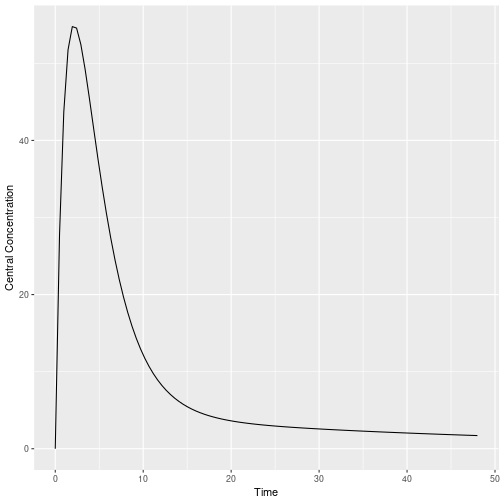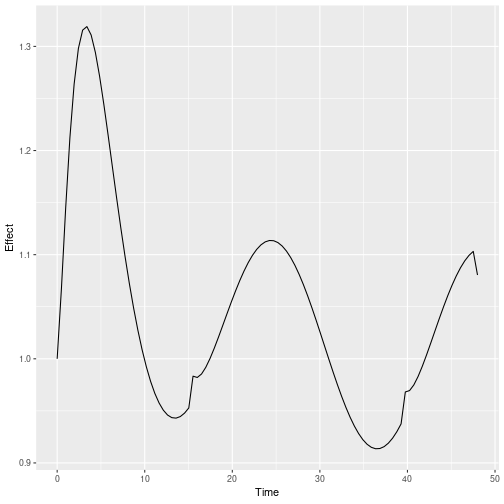<!–html_preserve–><!–/html_preserve–>

Covariates are easy to specify in RxODE, you can specify them as a variable. Time-varying covariates, like clock time in a circadian rhythm model, can also be used. Extending the indirect response model already discussed, we have:

``````library(RxODE)
mod3 <- RxODE({
KA=2.94E-01;
CL=1.86E+01;
V2=4.02E+01;
Q=1.05E+01;
V3=2.97E+02;
Kin0=1;
Kout=1;
EC50=200;
## The linCmt() picks up the variables from above
C2   = linCmt();
Tz= 8
amp=0.1
eff(0) = 1  ## This specifies that the effect compartment starts at 1.
## Kin changes based on time of day (like cortosol)
Kin =   Kin0 +amp *cos(2*pi*(ctime-Tz)/24)
d/dt(eff) =  Kin - Kout*(1-C2/(EC50+C2))*eff;
})

ev <- eventTable(amount.units="mg", time.units="hours") %>%

## Create data frame of  8 am dosing for the first dose
cov.df  <- data.frame(ctime =(seq(0,48,length.out=100)+8) %% 24);
``````

Now there is a covariate present, the system can be solved using the cov option

``````r1 <- solve(mod3, ev, covs=cov.df,covs_interpolation="linear")
rxHtml(r1)
``````

Solved RxODE object
Parameters (\$params):
KA CL V2 Q V3 Kin0 Kout EC50 Tz amp pi
0.294 18.6 40.2 10.5 297 1 1 200 8 0.1 3.141593
Covariates (\$covs):
ctime
8.000000
8.484849
8.969697
9.454546
9.939394
10.424242
Initial Conditions ( \$inits):
eff
1
First part of data (object):
time C2 Kin eff
0.0000000 0.00000 1.099195 1.000000
0.4848485 27.76574 1.096795 1.067175
0.9696970 43.67518 1.092837 1.144556
1.4545455 51.75572 1.087385 1.212373
1.9393939 54.76289 1.080527 1.263980
2.4242424 54.57072 1.072373 1.298023

When solving ODE equations, the solver may sample times outside of the data. When this happens, this ODE solver uses linear interpolation between the covariate values. This is the default value. It is equivalent to R's `approxfun` with `method="linear"`, which is the default `approxfun`.

``````library(ggplot2)
ggplot(r1,aes(time,C2)) + geom_line() + ylab("Central Concentration") + xlab("Time");
````````````ggplot(r1,aes(time,eff)) + geom_line() + ylab("Effect") + xlab("Time");
``````Note that the linear approximation in this case leads to some kinks in the solved system at 24-hours where the covariate has a linear interpolation between near 24 and near 0.

In RxODE, covariate interpolation can also be the last observation carried forward, or constant approximation. This is equivalent to R's `approxfun` with `method="constant"`.

r“{r,results="asis”} r2 <- solve(mod3, ev, covs=cov.df,covs_interpolation=“constant”) rxHtml(r2)

``````
which gives the following plots:

```r
ggplot(r1,aes(time,C2)) + geom_line() + ylab("Central Concentration") + xlab("Time");
````````````ggplot(r1,aes(time,eff)) + geom_line() + ylab("Effect") + xlab("Time");
``````In this case, the plots seem to be smoother.

You can also use NONMEM's preferred interpolation style of next observation carried backward (NOCB): eeee

``````r2 <- solve(mod3, ev, covs=cov.df,covs_interpolation="nocb")
rxHtml(r2)
``````

Solved RxODE object
Parameters (\$params):
KA CL V2 Q V3 Kin0 Kout EC50 Tz amp pi
0.294 18.6 40.2 10.5 297 1 1 200 8 0.1 3.141593
Covariates (\$covs):
ctime
8.000000
8.484849
8.969697
9.454546
9.939394
10.424242
Initial Conditions ( \$inits):
eff
1
First part of data (object):
time C2 Kin eff
0.0000000 0.00000 1.099195 1.000000
0.4848485 27.76574 1.096795 1.066686
0.9696970 43.67518 1.092837 1.143446
1.4545455 51.75572 1.087385 1.210549
1.9393939 54.76289 1.080527 1.261397
2.4242424 54.57072 1.072373 1.294675

which gives the following plots:

``````ggplot(r1,aes(time,C2)) + geom_line() + ylab("Central Concentration") + xlab("Time");
````````````ggplot(r1,aes(time,eff)) + geom_line() + ylab("Effect") + xlab("Time");
``````